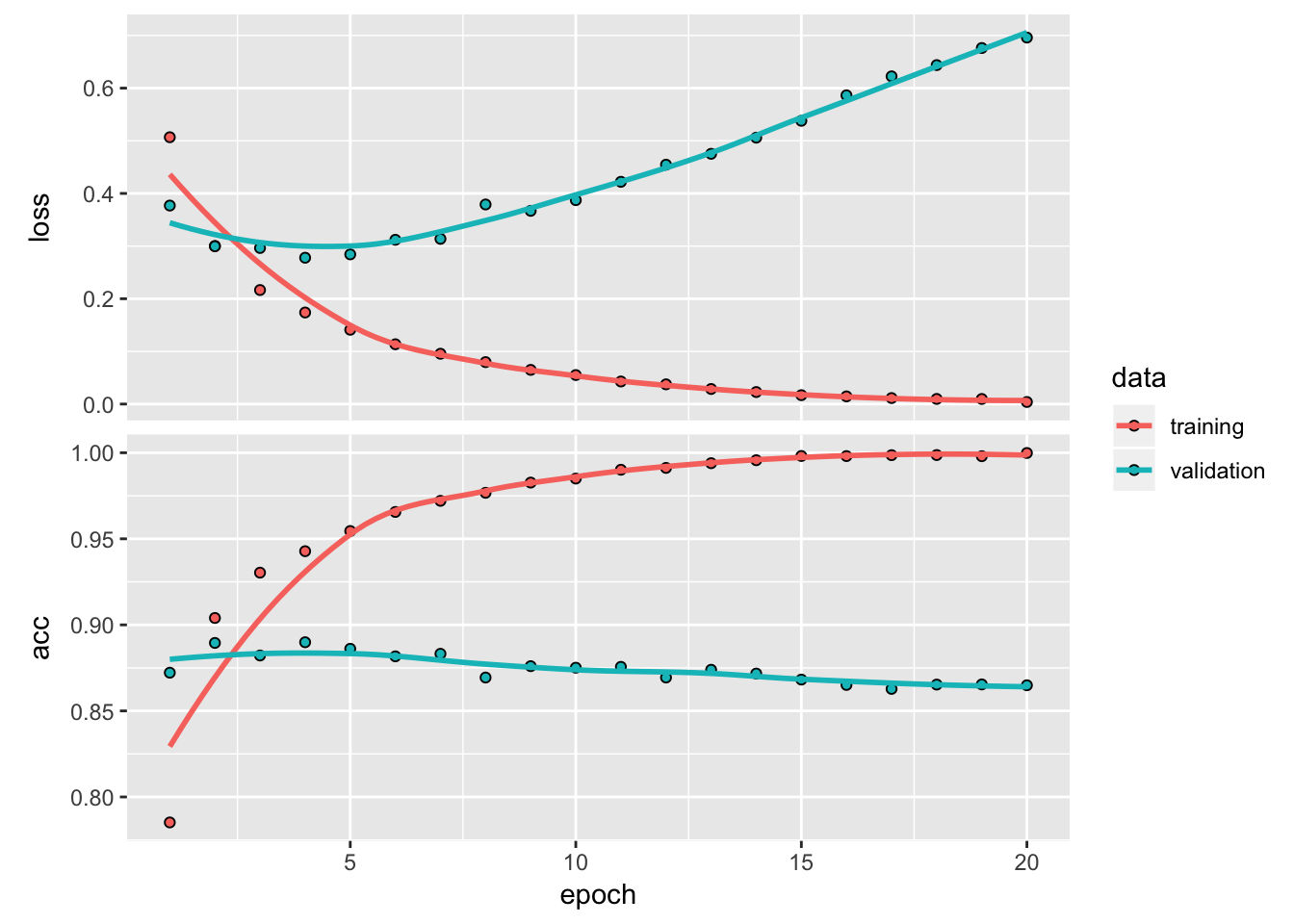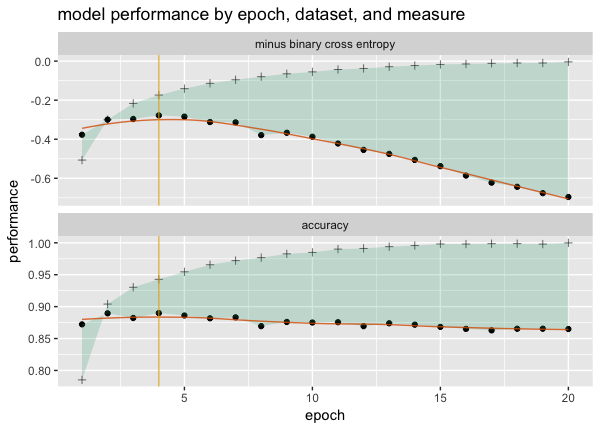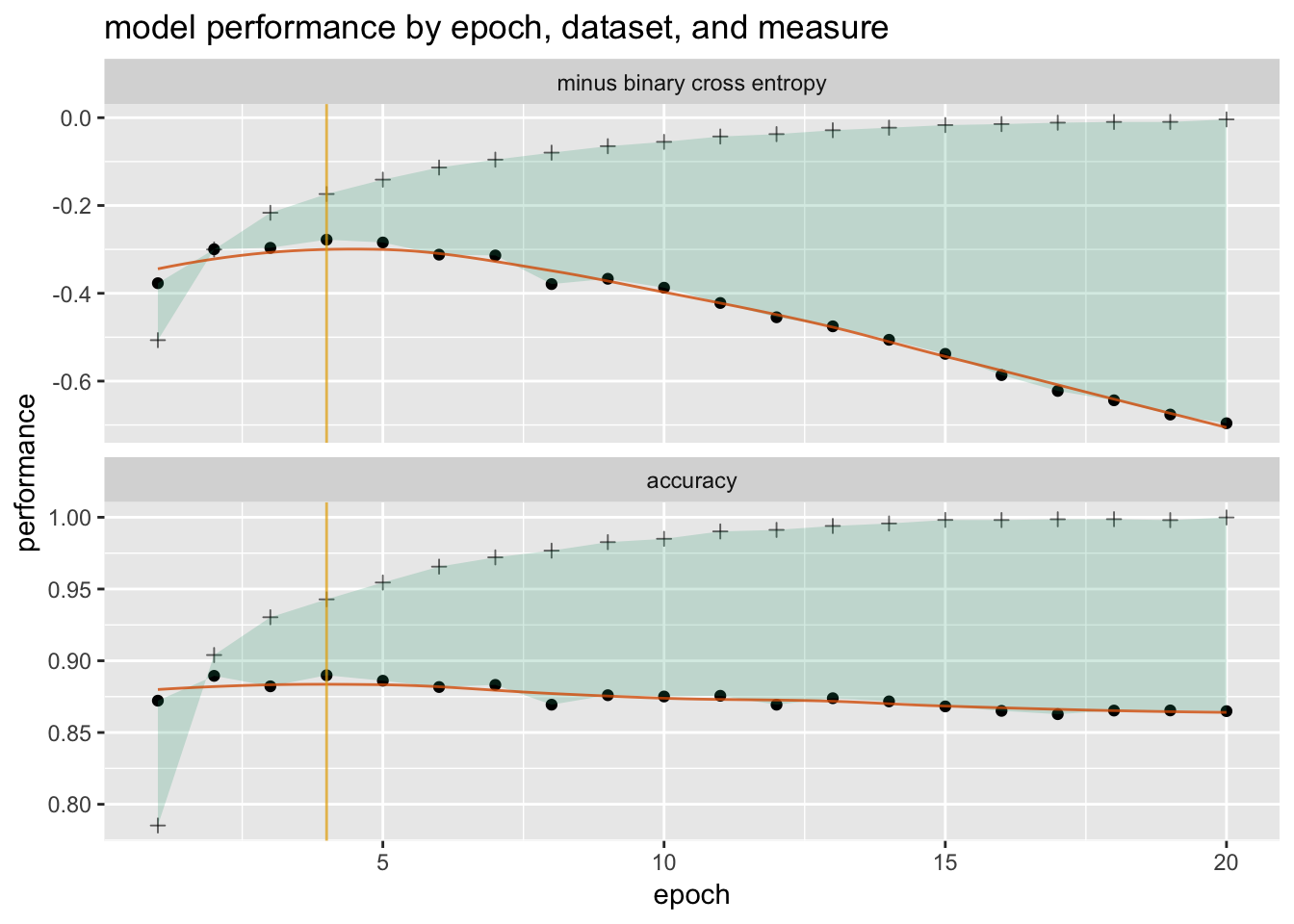# Fluid data reshaping with cdata

### Beyond pivot and unpivot

#### 2018-10-12

One of the principles of fluid data is that data layout (the shape: whether data is organized in rows, columns, or even separate tables) is separate from data semantics (which items of data logically belong together). A given task is most easily accomplished with a given data layout, so a single workflow with multiple tasks may require multiple data shapes. You should be able to effortlessly transform your data from one shape to another in order to efficiently execute your workflow.

Two of the most common data reshaping operations are pivot (also called tidyr::spread) and unpivot (also called tidyr::gather). While these two operations cover a large portion of typical data reshaping needs, some tasks will require more complex transformations. In this article we demonstrate data reshaping beyond pivot and unpivot with the cdata package.

## An Example

I’ve been playing recently with Keras in R. One of the things that I like in the R interface to Keras is how easy it is to visualize the training progress. The keras::fit function produces a history object that records the change in optimization loss and model performance by training epoch1 The gbm package for gradient boosting machines produces a similar graph of model performance by number of trees..

Here’s a Keras history plot, based on the example in the Keras in R documentation).This is a useful graph to help you determine the optimal number of epochs to train the model, but there are a few changes that I would like to make for myself:

• For perceptual ease, I’d like both graphs to show improvement in the same direction, say “up is better”.

• I would like the validation curves to perceptually dominate the training curves.

• I’d like the graph to identify the optimal stopping point2 For the sake of this article, we’ll call the optimal stopping point the epoch with the minimum validation loss..

One such graph (certainly not the only one) is a graph similar to one that John Mount introduced in a previous article:Let’s take the data from the Keras history object (the output of fit) and produce this graph in ggplot2. Here’s the history data, directly from fit()$metric: The data from Keras val_loss val_acc loss acc 0.3769818 0.8722 0.5067290 0.7852000 0.2996994 0.8895 0.3002033 0.9040000 0.2963943 0.8822 0.2165675 0.9303333 0.2779052 0.8899 0.1738829 0.9428000 0.2842501 0.8861 0.1410933 0.9545333 0.3119754 0.8817 0.1135626 0.9656000 We’ll add an epoch column and flip the loss so that larger is better: The starting data shape val_loss val_acc loss acc epoch -0.3769818 0.8722 -0.5067290 0.7852000 1 -0.2996994 0.8895 -0.3002033 0.9040000 2 -0.2963943 0.8822 -0.2165675 0.9303333 3 -0.2779052 0.8899 -0.1738829 0.9428000 4 -0.2842501 0.8861 -0.1410933 0.9545333 5 -0.3119754 0.8817 -0.1135626 0.9656000 6 We’ll refer to this as the starting data shape. This “wide” data shape is probably the easiest for Keras to produce, but it’s not the best shape for graphing (at least not in ggplot2). For our desired graph we want a geom_ribbon between the training and validation scores. This means we need the training and validation scores for a given epoch to be in the same row. We also want to facet_wrap the graph on the type of score (accuracy or loss). This means we need the flipped loss and the accuracy for a given epoch to be in different rows. We can draw a table that describes the shape we need and how it relates to our starting data shape: The data shape we need for plotting measure training validation minus binary cross entropy loss val_loss accuracy acc val_acc The interior of the table (shaded yellow) specifies the names of the original columns. The header of the table gives the names of the columns of the reshaped data: one column for the training scores, one for the validation scores, and a key column called measure that specifies whether a row reports the accuracy or the negated binary cross entropy. Notice that transforming the data from the starting shape to this shape cannot be done in a single spread or gather. In the rest of this article, we will show how we can do general reshaping transformations like this one with a single cdata call. ## Row Records and Blocks Our example data comes grouped by epoch. Let’s call the data for a single epoch a record. In the starting data shape, a record is a single row. We’ll call that shape a row record (“rowrec” for short). For plotting, we want the information from a single record distributed across multiple rows, as the table above (the control table) describes. We’ll call that shape a block. In cdata the control table specifies how to transform data from row records to blocks. Let’s work through this with a table that contains a single row record. onerow = startingData %.>% head(., n=1) A single-record table val_loss val_acc loss acc epoch -0.3769818 0.8722 -0.506729 0.7852 1 First, create the control table. controlTable = build_frame( "measure" , "training", "validation" | "minus binary cross entropy", "loss" , "val_loss" | "accuracy" , "acc" , "val_acc" ) The control table measure training validation minus binary cross entropy loss val_loss accuracy acc val_acc The function cdata::rowrecs_to_blocks takes data organized as row records, and reorganizes it into blocks. It takes as input the data, the control table, and an additional optional argument called columnsToCopy for columns that you want to carry along. One such column is often the record id (in this case, epoch). library("cdata") blockf = rowrecs_to_blocks(onerow, controlTable=controlTable, columnsToCopy = 'epoch')  A record distributed into a block of rows epoch measure training validation 1 minus binary cross entropy -0.506729 -0.3769818 1 accuracy 0.785200 0.8722000 The function cdata::blocks_to_rowrecs takes data organized as blocks and reshapes it into row records. It takes as input the data, the control table, and an additional required argument keyColumns that designates the record or group id (again, in this case, epoch)3 You can pass keyColumns a vector of column names, in the case of composite keys. There is also an optional columnsToCopy argument.. rowf = blocks_to_rowrecs(blockf, controlTable=controlTable, keyColumns='epoch')  A record as a single rowrec epoch acc loss val_acc val_loss 1 0.7852 -0.506729 0.8722 -0.3769818 A neat thing about the cdata functions is that you can use them on the control table itself! This is a useful way to check that you have specified your reshaping correctly. Let’s move the control table into a single row. cR = controlTable %.>% blocks_to_rowrecs( ., controlTable = controlTable, # no record id column keyColumns = NULL) %.>% # reorder the columns as in the original shape select_columns(., c("val_loss", "val_acc", "loss", "acc")) The control table reshaped to a single row val_loss val_acc loss acc val_loss val_acc loss acc Now move the transformed table back into its original shape. cT = cR %.>% rowrecs_to_blocks( ., controlTable = controlTable) The control table back in its original shape measure training validation minus binary cross entropy loss val_loss accuracy acc val_acc ## Back to the Example Now let’s apply the control table to all the data. dToPlot = rowrecs_to_blocks( startingData, controlTable = controlTable, columnsToCopy = "epoch") The first few rows of the reshaped data epoch measure training validation 1 minus binary cross entropy -0.5067290 -0.3769818 1 accuracy 0.7852000 0.8722000 2 minus binary cross entropy -0.3002033 -0.2996994 2 accuracy 0.9040000 0.8895000 3 minus binary cross entropy -0.2165675 -0.2963943 3 accuracy 0.9303333 0.8822000 And from here we can create the desired plot. # find the optimal stopping point pick = which.max(startingData$val_loss)

# reorder the graphs
dToPlot$measure = factor(dToPlot$measure,
levels = c("minus binary cross entropy",
"accuracy"))
ggplot(data = dToPlot,
aes(x = epoch,
y = validation,
ymin = validation,
ymax = training)) +
geom_point() +
geom_point(aes(y = training),
shape = 3, alpha = 0.6) +
geom_ribbon(alpha = 0.2, fill = "#1b9e77") +
stat_smooth(geom = "line",
se = FALSE,
color  = "#d95f02",
alpha = 0.8,
method = "loess") +
geom_vline(xintercept = pick,
alpha=0.7, color = '#e6ab02') +
facet_wrap(~measure, ncol = 1,
scales = 'free_y') +
ylab("performance") +
ggtitle("model performance by epoch, dataset, and measure")Voilà!

## Pivot and Unpivot

Now that we’ve distinguished data semantics (records) from data layout (row records and blocks), it’s easy to see that pivot and unpivot are special cases. Unpivot (or tidyr::gather) transforms a row record (where the column names of the data frame are the keys that identify the data items) into a block shaped as a single column, plus an additional column of keys. Pivot (or tidyr::spread) transforms a column block and its corresponding key column into a row record.

You can use blocks_to_rowrecs and rowrecs_to_blocks to pivot and unpivot data, but because these two transformations are so common, cdata has special case functions pivot_to_rowrecs and unpivot_to_blocks that don’t require you to explicitly create the control table. See our article Coordinatized Data: A Fluid Data Specification for examples.

## Conclusion

Separating important data semantics from inessential details of data layout helps to free our minds from conflating what we want from how to achieve it. In an ideal world, you should select the appropriate algorithm, procedure, or visualization based on what best fits your needs, not on which procedure is the least hassle to implement for data in a given layout. cdata aims to take some of the hassle out of data reshaping so you can focus on your actual goals.